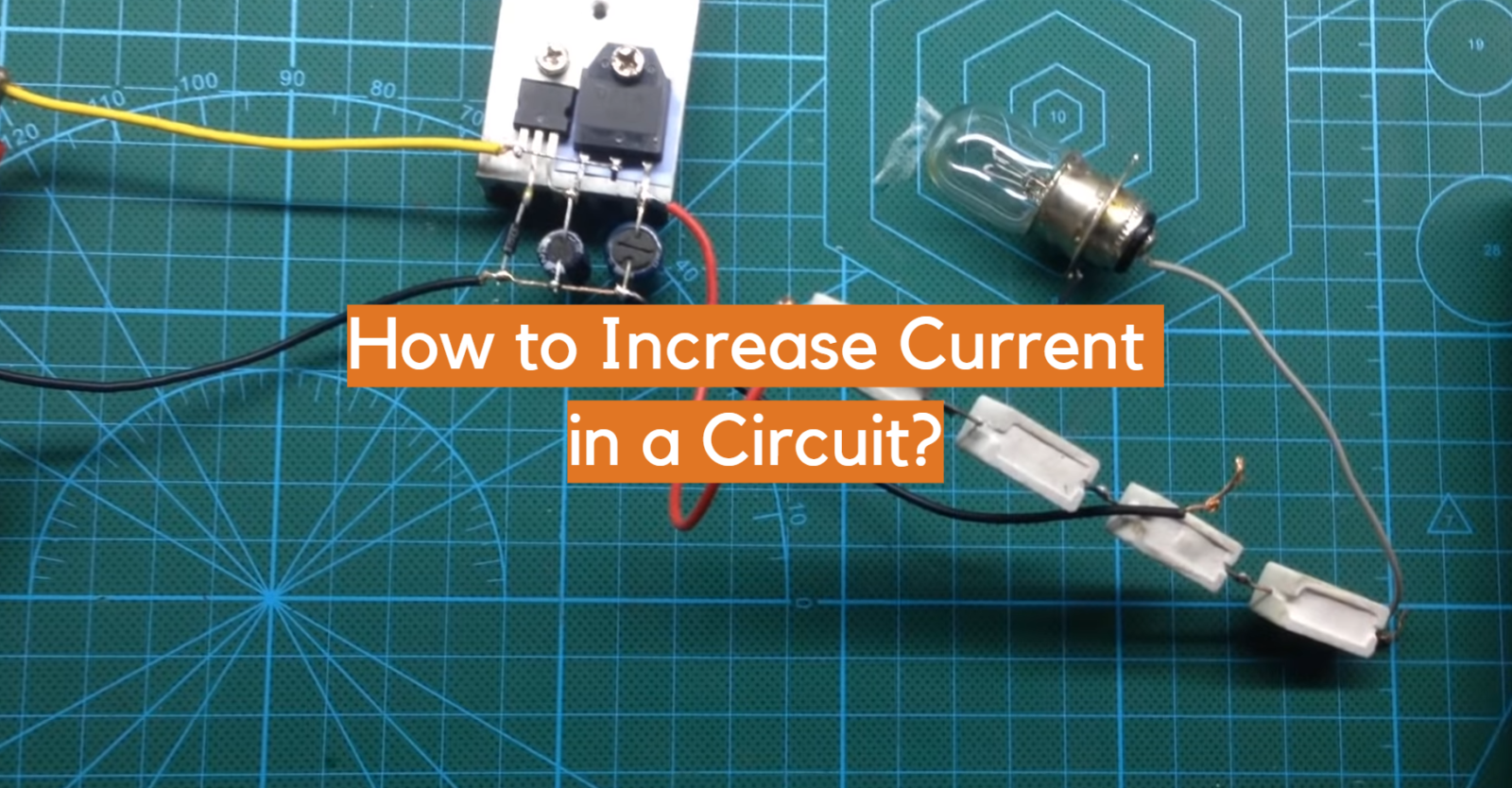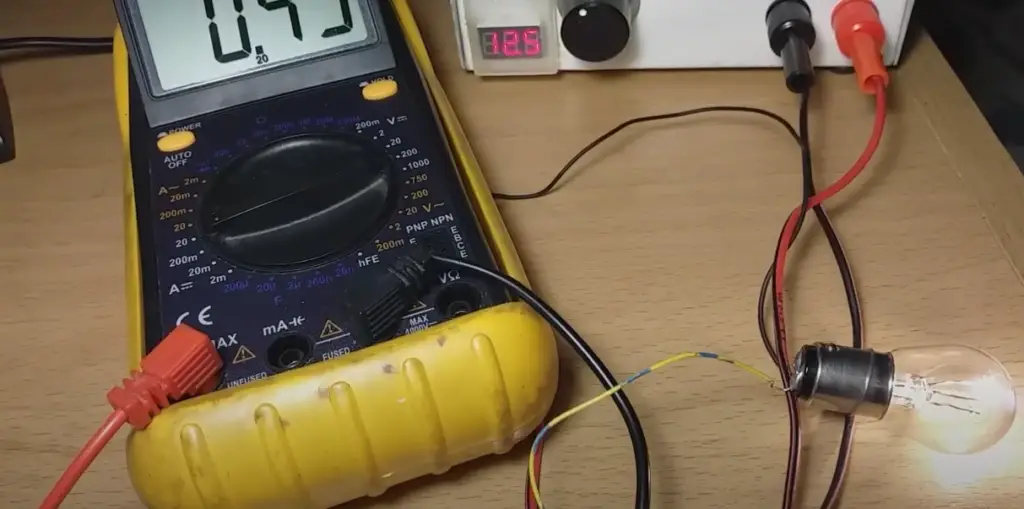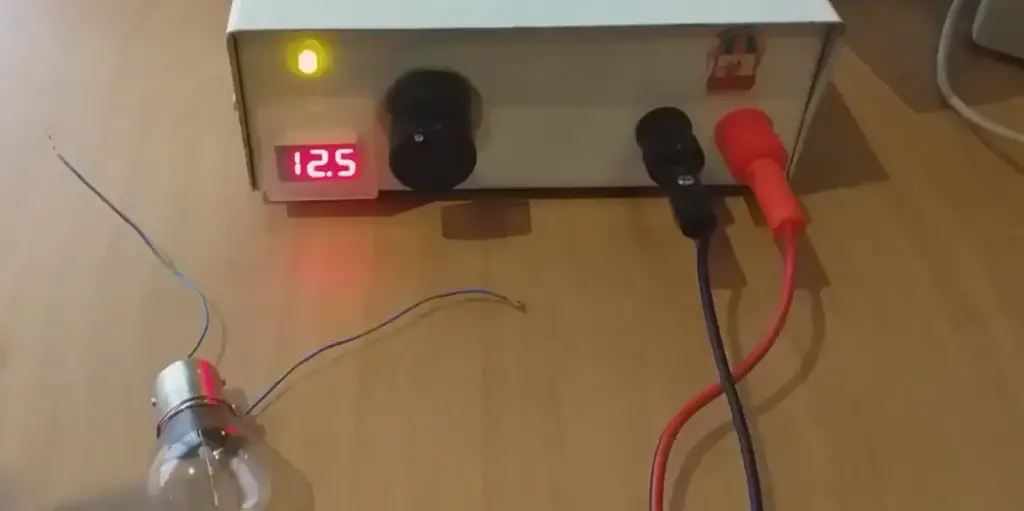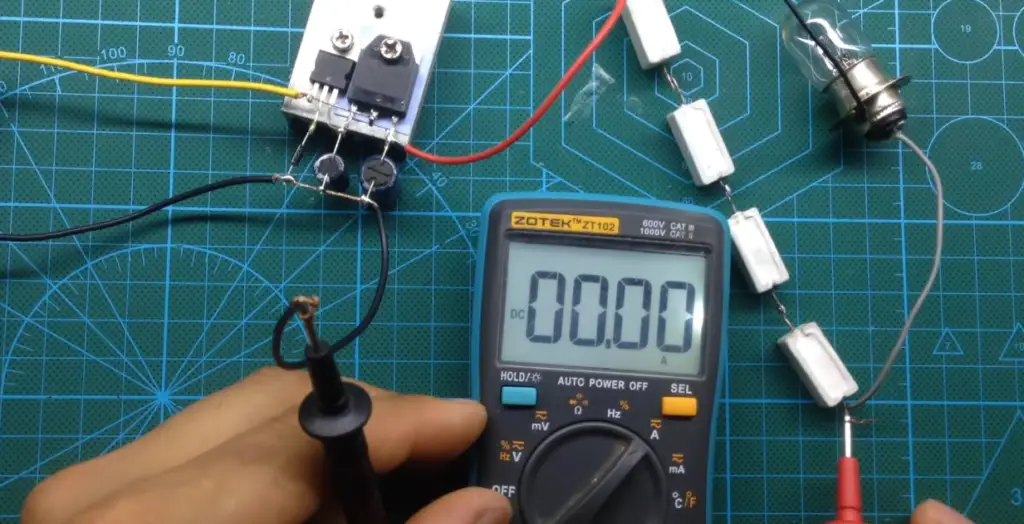# How to Increase Current in a Circuit?Do you want to learn how to increase current in a circuit? You’ve come to the right place! In this article, we will discuss some common methods that can help you boost the current in your electrical circuits. We’ll also provide some basic electronics trivia and tips to keep in mind while working with circuits. So whether you’re a beginner or an experienced electrician, read on for all the information you need to know about increasing current in a circuit!

## Currents and Their Role in a Circuit

An electrical current is the flow of electrons through a conductor, such as a metal wire.

The SI unit for current is the ampere (A), and it is represented by the letter I.

The rate at which charge flows is called electric potential difference or voltage and is measured in volts (V). Potential difference can be thought of as water pressure; it pushes electrons around a circuit.

You can think of current as the amount of water flowing past a point in a given time. If you have ever seen water flowing in a river, you will know that the further away from the source you are, the slower the water flows. This is because there are more and more molecules bumping into each other, slowing down the overall flow. The same thing happens with electrons in a circuit: the further they have to travel, the more collisions they experience, and the slower the current.Current plays a vital role in the proper functioning of an electrical circuit. It is responsible for providing the necessary power to operate devices connected to the circuit. If the current in a circuit is too low, it can cause devices to malfunction or stop working altogether. Conversely, if the current is too high, it can damage devices and pose a safety hazard. Therefore, it is important to ensure that the current in a circuit is within the proper range. 

## What Defines a Current?

There are several factors that can affect the amount of current flowing through a circuit.

One of the most common is resistance. Resistance occurs when electrons encounter atoms or molecules that they cannot pass through easily. This impedes the flow of electrons and increases resistance. Other factors that can increase resistance include the length of the circuit and the cross-sectional area of the conductor. The higher the resistance, the more the material opposes the current. When you increase the resistance in a circuit, it takes more voltage to push the same amount of current through it.

Another factor that can affect current is voltage. Voltage is the force that drives electrons through a circuit. Both voltage and current are the two basic properties of an electrical circuit.

Current is a measure of the flow of electrons through the circuit, while voltage is a measure of the potential difference between two points in that circuit.

To put it simply, voltage can be thought of as the pressure of the electrons in a circuit, while current is the flow of those electrons. The higher the voltage, the more forcefully electrons will flow through the circuit.There are two main ways to apply voltage: using a battery or using an AC power source. Batteries are the most common way to apply voltage in small electronic devices like flashlights and cell phones. AC power sources are more common in larger devices like TVs and refrigerators. , 

## When Would You Need to Increase Current in a Circuit?

There are a few reasons you might need to increase current in your circuit. Maybe your project requires more power than what’s available from the standard voltage sources. Or maybe you’re trying to minimize heat loss by using a lower voltage supply but still need more current than what that can provide. Whatever the reason, there are a few ways to go about increasing current in your circuit.

## Ways to Increase Current in a Circuit

Now that we know what current is, let’s move on to how to increase it in a circuit. In this section we will discuss the two main methods!

### Ohms Law in General

Now that we know how to calculate current, let’s talk about how to increase it. The first step is understanding Ohm’s law, which states that the current in a circuit is equal to the voltage divided by the resistance. This means that if you want to increase the current in a circuit, you can either increase the voltage or decrease the resistance.

Ohm’s law is the most important equation in all of electrical engineering. It relates voltage, current, and resistance:

V=IR

Where V is voltage in volts, I is current in amps, and R is resistance in ohms. If you know any two of those values, you can always calculate the third.
For example, if you have a resistor with a resistance of 1000 ohms (R=1000), and you apply one volt to it (V=1000), then the current through that resistor will be one milliamp (.001 amps).

And from this we can conclude that an alteration in any of these three values will directly affect the other two. So, if you want to increase the current in a circuit, you need to increase either the voltage or decrease the resistance. , , 

### By increasing the applied voltage

The most common method of increasing current is by increasing the applied voltage. If we take our previous example and increase the voltage to two volts, then the current will become two milliamps (.002 amps). The amperage in circuit can be changed if the resistance stays the same and the voltage is increased.

This is a simple way to increase current, but it has its limitations. The main limitation is that you can only increase the voltage so much before you start damaging your components. Every component has a maximum voltage that it can withstand before it breaks down. This is known as the breakdown voltage.

For example, if you have a resistor with a maximum voltage of 100 volts (Vmax=100), and you apply 150 volts to it (V=150), then the resistor will break and the current will go to infinity.

Therefore, it is important to always check the maximum voltage of your components before applying a voltage to them.

Another limitation of increasing voltage is that it will also increase the power dissipation in your circuit. Power dissipation is the amount of heat that is generated by the components in a circuit.

Increasing voltage can be done in two ways. The first way is by using a higher voltage power supply. The second way is by adding batteries in series to increase the voltage.

When the voltage is increased, the electrons will flow more forcefully through the circuit, increasing the current. However, as we just explained this method also has its drawbacks. Increasing the voltage can also cause damage to devices and pose a safety hazard. Therefore, it is important to use caution when increasing voltage and only do so if absolutely necessary. , , , 

### By decreasing resistance

If the applied voltage is held constant, then the only way to increase current is by decreasing resistance. Resistance is measured in ohms and it’s represented by the symbol Ω. As we mentioned before, resistance occurs when electrons encounter atoms or molecules that they cannot pass through easily. This impedes the flow of electrons and increases resistance. , , 

Resistance can be influenced in a number of ways.

### Material

The first way to decrease resistance is by using a material with low resistivity.

The resistivity of a material is how resistant it is to the flow of electrons.

This property is intrinsic to the material and cannot be changed. Some materials have high resistivity like glass, while others have low resistivity like copper.

If you want to decrease the resistance in your circuit, you need to use a material with low resistivity like copper or aluminum. 

### Wire length

The second way to decrease resistance is by using a shorter wire. The longer the wire, the greater the resistance because there are more atoms for the electrons to encounter. This is why wires are often made as short as possible. , 

### Wire thickness

The thickness of the wire also affects resistance. A thicker wire with small diameter has less room for the electrons to flow and thus has less resistance. This is why wires with bigger diameter are often used when high currents are required. You can think of it like a water pipe. A wider pipe can carry more water than a narrow pipe because there is more space for the water to flow. The same is true for electricity and wires. , 

### Number of lamps in series

Another way to decrease resistance is by adding more lamps in series. When you have more lamps in a circuit, the overall resistance of the circuit increases because each lamp adds its own resistance to the circuit.

The more lamps there are in the circuit, the less current flows because the electrons have more obstacles (lamps) to encounter. 

### Switching to a lower-rated resistor

One sure way to decrease resistance is to simply switch to a lower-rated resistor. This will have an immediate effect on the current, as long as the voltage remains constant.

For example, if you have a 4 ohm resistor in your circuit and you want to increase the current, you can replace it with a 2 ohm resistor. This will effectively halve the amount of resistance in your circuit and double the current.

However, be careful not to go too low or you may end up damaging your components. It’s always best to err on the side of caution when working with electricity!

If you’re not sure what resistor to use, there are online calculators that can help you determine the correct value for your circuit.

You can also use a variable resistor. A variable resistor is also known as a potentiometer, and it’s a type of resistor that can be manually adjusted.

Variable resistors are often used to control the amount of current in a circuit. For example, if you have an LED that only lights up when a certain amount of current is flowing through it, then you can use a potentiometer to adjust the current until the LED lights up.Potentiometers are not the only type of variable resistor. There are also rheostats and trimpots. Rheostats are used to control larger currents, while trimpots are used to make small adjustments. , 

### Using resistors in parallel

Another way to decrease resistance is by using resistors in parallel. A resistor is a device that is used to create resistance in a circuit. Resistors are made of materials that have a high resistance, such as carbon or metal oxide.

When resistors are placed in parallel, they create multiple paths for the current to flow through. This increases the amount of current because there are more paths for the electrons to take. 

## FAQ

### What causes low current?

There can be many reasons for low current in a circuit. The most common reason is resistance. Anytime there is resistance in the circuit, it will cause the current to drop. Other causes can include poor contact between wires, or a break in the circuit.

Another common reason for low current is voltage drop. Voltage drop occurs when the voltage across a component drops below the ideal voltage. This can happen when the load on the circuit is too high, or if there are problems with the power supply.

Another possibility is that the current limit has been reached. The current limit is the maximum amount of current that can flow through a given component without damaging it.

### Can you increase current without increasing voltage?

There are ways to increase current without necessarily increasing voltage. One way to do this is by decreasing the resistance in the circuit. This can be done by adding more conductors in parallel or by using a material with a lower resistivity. Another way to increase current is to add energy to the system, either by increasing the voltage or by adding power directly to the current.

### What would increase the current?

The current in a circuit can be increased by increasing the voltage or by decreasing the resistance. Another way to increase current is to use a material with a lower resistivity, such as copper instead of aluminum.

It is important to note that increasing the current too much can cause damage to components or start fires, so care must be taken when making these changes.

### What increases current in a wire?

The current in a wire is increased by the number of electrons passing through the wire per second. This can be increased by either increasing the voltage or decreasing the resistance.

It’s important to know that you can’t just increase the current in a circuit without changing something else.

For example, if you tried to increase the current in your car’s engine by adding more gasoline, it would just explode. The same goes for circuits – if you try to push too much current through a wire, it will heat up and potentially catch fire.

### What is one way to increase the current in a wire?

One way to increase the current in a wire is to increase the voltage. This will cause more electrons to flow through the wire. Another way to increase the current is to decrease the resistance. This will allow more electrons to flow through the wire. Finally, you can also increase the cross-sectional area of the wire. This will allow more electrons to flow through the wire because there is less resistance for them to overcome.

## Conclusion

Increasing the current in a circuit can be done by increasing the voltage or decreasing the resistance. If you want to increase the current without affecting the other parts of the circuit, you can add a second battery in parallel or use a more powerful one. You could also decrease the resistance by using thinner wires or decreasing the wire length. Ohm’s law is your best friend when it comes to troubleshooting current issues in a circuit.

Just keep in mind that increasing the voltage comes with its own dangers, so be sure to take the necessary precautions. If you’re ever unsure, it’s always best to consult with a professional.

Do you have any other tips on how to increase current in a circuit? Let us know in the comments below! Thanks for reading!